## Practical use of Unity3d engine.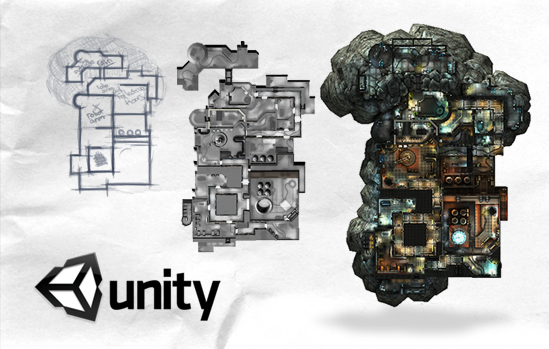## Rotate, pan and zoom of object

Contents
• Introduction;
• Task #1: Rotate, pan and zoom;
• Scene creation;
• Rotate, pan and zoom of object;

Introduction
Hi! This is the first article about development in Unity3d. In my articles, I will try to describe most common application development tasks in Unity3d and their solutions.

#### Task #1: Rotate, pan and zoom.

Now let us take a look to task: we need to show model of “flat”. This object user can rotate, pan and zoom.

Scene creation
At first, we will create flat. It’s a model that will be created from simple boxes. First of all, create an empty scene. To do this, select the menu item “File/New Scene” as show on fig. 1.1.Fig. 1.1. – Creation of new scene

As a result we get empty scene, shown in fig. 1.2.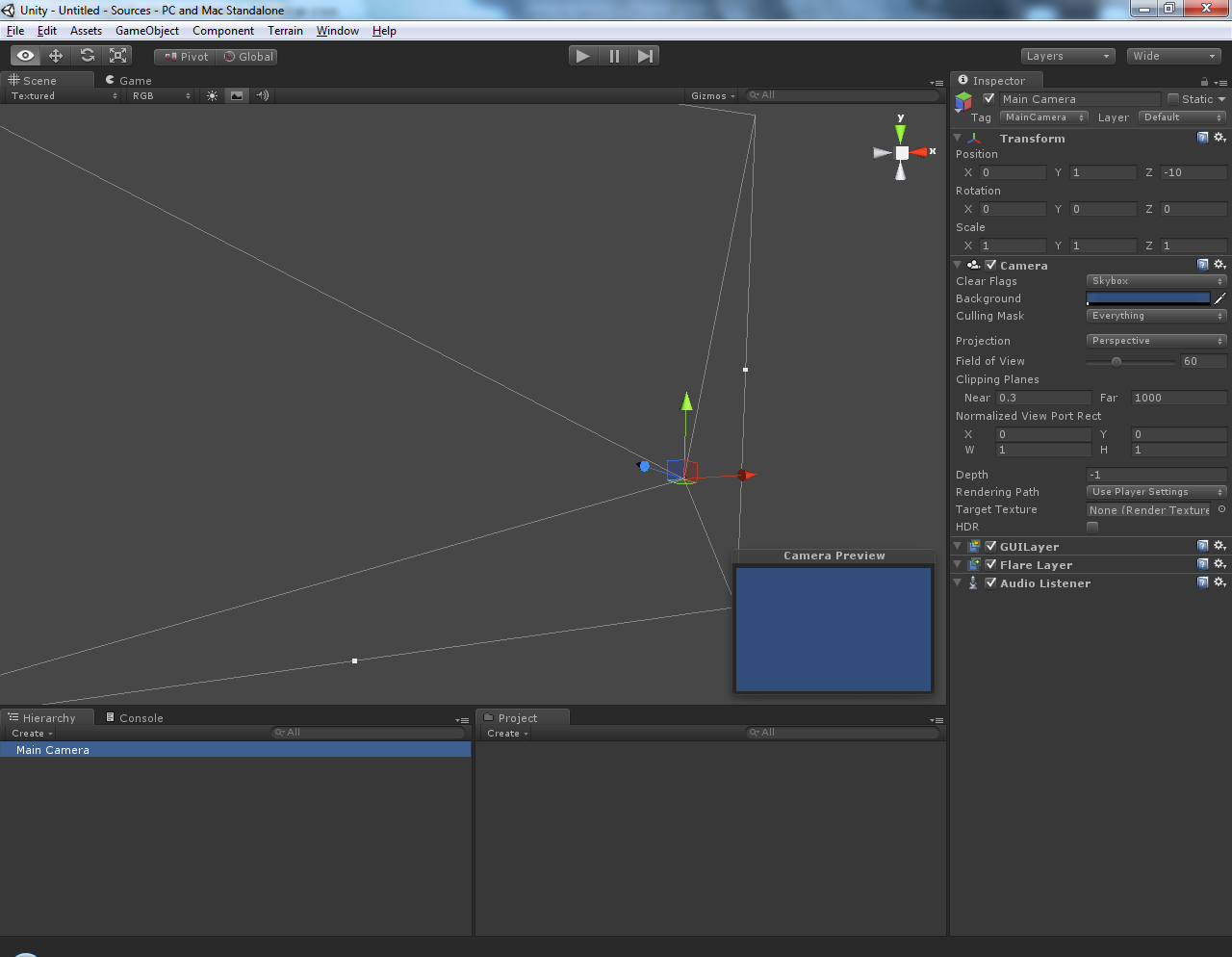Fig. 1.2. – Empty scene in Unity3d

Now, let’s create “floor” of the flat. Choose menu item “GameObject/CreateOther/Cube” (fig.1.3).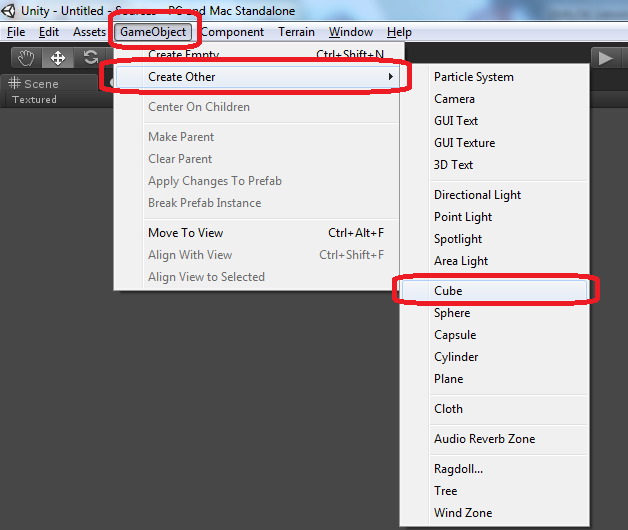Fig 1.3. – How to create cube in Unity3d

We can create “floor” by scaling this cube (Fig. 1.4).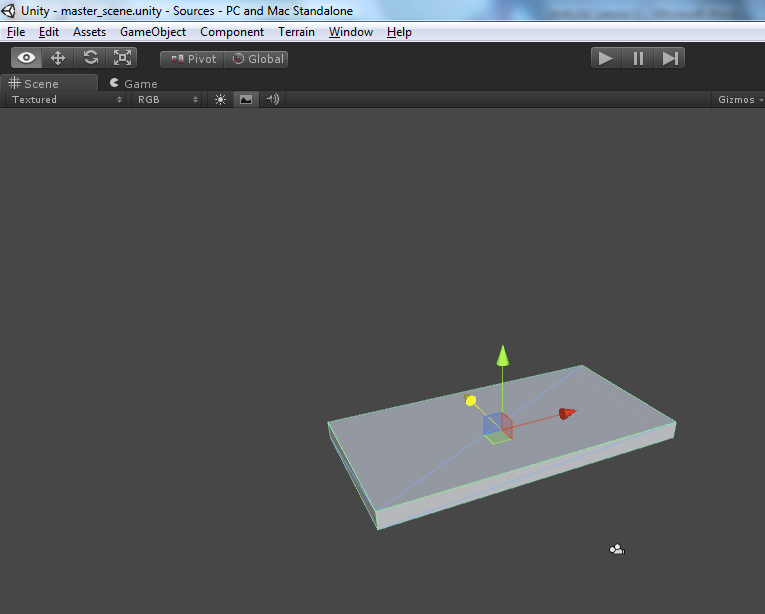Fig 1.4. – Created “floor” of the room

Change name of the “floor” in «Hierarchy» window. Let’s name it «Floor». The process of changing the name of the object is shown on fig. 1.5.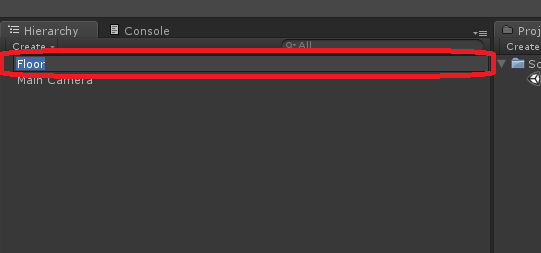Fig 1.5. – The process of changing the name of the object.

Now, let’s create “rooms” using same cubes. Also add to scene a light. After that, add an empty object to the scene. Name of this object is “flat”. Set “flat” as parent to floor, rooms and light. As a result we can get scene, shown in fig. 1.6.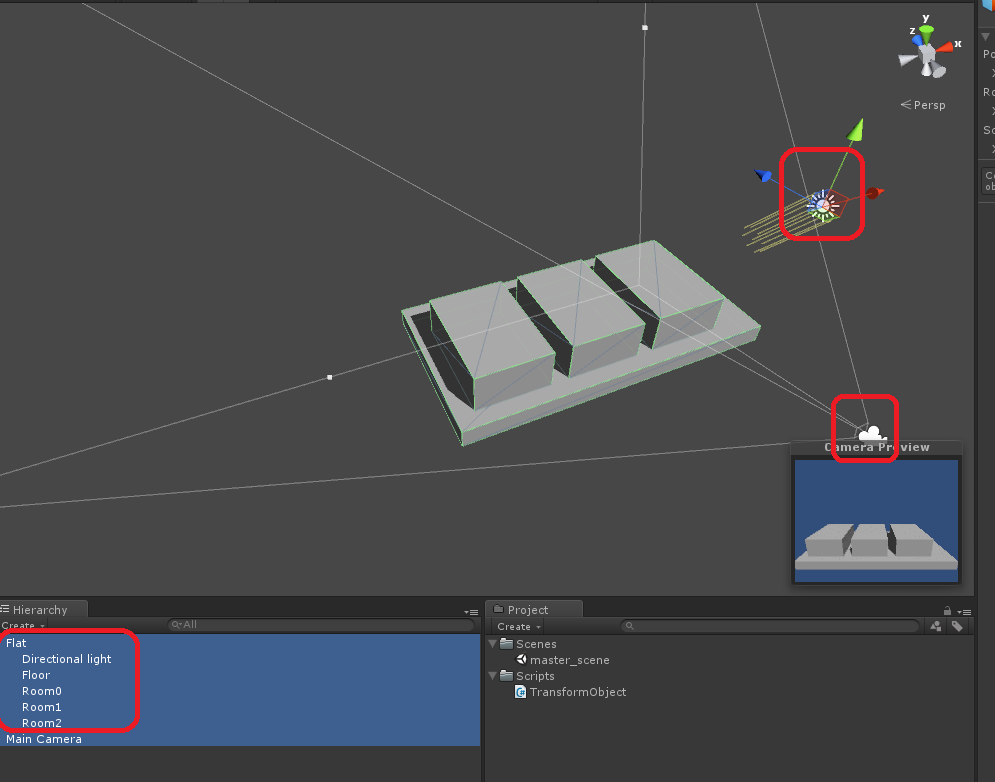Fig 1.6 – Scene that contains 3 rooms, floor, light and camera

Please note that rooms are created as a child to flat. We can see this in “Hierarchy” window at fig. 1.6.

#### Rotate, pan and zoom of object

There are a lot of ready scripts in the internet today that provides rotate, pan and zoom implementation. But, if we want clearly understand implementation of object manipulation, I suggest to use script “TransformObject.cs”. This script is well commented, but I’ll do some description.
Usually on the internet you can find a set of scripts, that you can just assign to object at hierarchy, and it will work in parallel with set of other scripts. Unity3d editor allows you to assign scripts to any amount of objects, and these scripts will work parallel. But I prefer to develop applications that have only one entry point. How properly use ability to assign additional scripts to objects, I will tell you in future articles.
So, here is the algorithm of using script “TransformObject”:
Create class «AppRoot». AppRoot – it is an entry point of our application. Create an instance of the TransformObject object in AppRoot. In function «AppRoot.Start» initialize it. Then, set rotate around object. This is the object around which the camera rotates. To do this, call method «SetTransformRotateAround».
```public class AppRoot : MonoBehaviour
{
private TransformObject mTransform; // TransformObject implements rotate / pan / zoom

private GameObject mGOFlat; // GO rotate around
private const string cGONameFlat = "Flat";

void Start()
{
// Find cGONameFlat in scene
mGOFlat = GameObject.Find(cGONameFlat);

// instantiate TransformObject and sets its rotate around object
mTransform = new TransformObject();
mTransform.SetTransformRotateAround(mGOFlat.transform);
}
void Update()
{
mTransform.Update();
}
}
```
I suggest using the class «TransformObject», because we will further add new functionality to it, for example for using it in Android and iOS devices.
So, here is «TransformObject» source code:
```
using UnityEngine;
using System;

public class TransformObject
{
///////////////////////////////////////////////////////////////////////////
#region Variables

// variables

#if UNITY_STANDALONE_WIN || UNITY_STANDALONE_OSX || UNITY_WEBPLAYER
private float RotationSpeed = 1500;
private float MoveSpeed = 10.0f;
private float ZoomSpeed = 15.3f;
#endif // UNITY_STANDALONE_WIN || UNITY_STANDALONE_OSX || UNITY_WEBPLAYER

#if UNITY_ANDROID || UNITY_IPHONE
private float RotationSpeed = 9.5f;
private float MoveSpeed = 1.09f;
private float ZoomSpeed = 0.009f;

private float mOldFingerDelta = 0;
private const float mFingerDistanceEpsilon = 1.0f;

#endif // UNITY_ANDROID || UNITY_IPHONE

public float MinDist = 2.0f;
public float MaxDist = 50.0f;

private Transform mMoveObject = null;

#endregion
///////////////////////////////////////////////////////////////////////////

///////////////////////////////////////////////////////////////////////////
#region Public methods

///
///
///
public TransformObject()
{
EnabledMoving = true;
}

///
/// Sets transform that will be used as "center" of the rotate / pan / zoom
///
public void SetTransformRotateAround(Transform goMove)
{
mMoveObject = goMove;
if (mMoveObject == null)
{
Debug.LogWarning("Error! Cannot find object!");
return;
}
}

public void Update()
{
if (!EnabledMoving)
{
return;
}

Vector3 dir = mMoveObject.position - Camera.main.transform.position;
float dist = Math.Abs(dir.magnitude);

Vector3 camDir = Camera.main.transform.forward;
Vector3 camLeft = Vector3.Cross(camDir, Vector3.down);
Vector3 camDown = Vector3.Cross(camDir, camLeft);
//Vector3 camUp = Vector3.Cross(camLeft, camDir);

#if UNITY_STANDALONE_WIN || UNITY_STANDALONE_OSX || UNITY_WEBPLAYER

float dx = Input.GetAxis("Mouse X");
float dy = Input.GetAxis("Mouse Y");

// rotate
if (Input.GetMouseButton(0))
{
mMoveObject.Rotate(camLeft, dy * RotationSpeed * Time.deltaTime, Space.World);
mMoveObject.Rotate(Vector3.down, dx * RotationSpeed * Time.deltaTime, Space.Self);
}

// move
if (Input.GetMouseButton(1))
{
Vector3 camPos = Camera.main.transform.position;
camPos += -camLeft * MoveSpeed * dx * Time.deltaTime;
camPos += -camDown * MoveSpeed * dy * Time.deltaTime;
Camera.main.transform.position = camPos;
}

// zoom
if (Input.GetAxis("Mouse ScrollWheel") > 0)
{
if (dist > MinDist)
{
mMoveObject.Translate(-dir * ZoomSpeed * Time.deltaTime, Space.World);
}
}

if (Input.GetAxis("Mouse ScrollWheel") < 0)
{
if (dist < MaxDist)
{
mMoveObject.Translate(dir * ZoomSpeed * Time.deltaTime, Space.World);
}
}

#endif // UNITY_STANDALONE_WIN || UNITY_STANDALONE_OSX || UNITY_WEBPLAYER

#if UNITY_ANDROID || UNITY_IPHONE

// rotate
if (Input.touchCount == 1)
{
mMoveObject.Rotate(camLeft, Input.touches.deltaPosition.y * RotationSpeed * Time.deltaTime, Space.World);
mMoveObject.Rotate(Vector3.down, Input.touches.deltaPosition.x * RotationSpeed * Time.deltaTime, Space.Self);
}

if (Input.touchCount == 2)
{
UnityEngine.Vector2 deltaFingerVec = Input.touches.position - Input.touches.position;

float deltaFingerValue =
Mathf.Sqrt(deltaFingerVec.x * deltaFingerVec.x + deltaFingerVec.y * deltaFingerVec.y);

// move
bool moved = false;
{
float moveX = 0;
float moveY = 0;

// moveX
if (Input.touches.deltaPosition.x < 0 && Input.touches.deltaPosition.x < 0)
{
moveX = Mathf.Max(Input.touches.deltaPosition.x, Input.touches.deltaPosition.x);
moved = true;
}
else if (Input.touches.deltaPosition.x > 0 && Input.touches.deltaPosition.x > 0)
{
moveX = Mathf.Min(Input.touches.deltaPosition.x, Input.touches.deltaPosition.x);
moved = true;
}

// moveY
if (Input.touches.deltaPosition.y < 0 && Input.touches.deltaPosition.y < 0)
{
moveY = Mathf.Max(Input.touches.deltaPosition.y, Input.touches.deltaPosition.y);
moved = true;
}
else if (Input.touches.deltaPosition.y > 0 && Input.touches.deltaPosition.y > 0)
{
moveY = Mathf.Min(Input.touches.deltaPosition.y, Input.touches.deltaPosition.y);
moved = true;
}

Vector3 camPos = Camera.main.transform.position;
camPos += -camLeft * MoveSpeed * moveX * Time.deltaTime;
camPos += -camDown * MoveSpeed * moveY * Time.deltaTime;
Camera.main.transform.position = camPos;
}

// zoom
if (!moved && Mathf.Abs(deltaFingerValue - mOldFingerDelta) > mFingerDistanceEpsilon)
{
if (deltaFingerValue - mOldFingerDelta > 0)
{
if (dist > MinDist)
{
mMoveObject.transform.Translate(-dir * ZoomSpeed * Time.deltaTime * deltaFingerValue, Space.World);
}
}

if (deltaFingerValue - mOldFingerDelta < 0)
{
if (dist < MaxDist)
{
mMoveObject.transform.Translate(dir * ZoomSpeed * Time.deltaTime * deltaFingerValue, Space.World);
}
}
}

mOldFingerDelta = deltaFingerValue;
}

#endif // UNITY_ANDROID || UNITY_IPHONE

}
#endregion
///////////////////////////////////////////////////////////////////////////

///////////////////////////////////////////////////////////////////////////
#region Properties
///
/// Gets or set value indicating if transformation is enabled
///
public bool EnabledMoving
{
get;
set;
}

///
/// Gets game object that moves around
///
public Transform MoveObject
{
get
{
return mMoveObject;
}
}

#endregion
///////////////////////////////////////////////////////////////////////////
}

```
And now, let us take a look at «TransformObject» class.
Parameter «EnabledMoving» determines whether a rotation, pan and zooming is processed.Function «SetTransformRotateAround» sets rotate around object. In case user pass null object, Unity3d will write error warning to log. User of the class «TransformObject» also should call «Update» function for properly using of this class. This function will do rotate, pan and zoom. Key moments of “Update” function:
Camera direction vector:
```Vector3 camDir = Camera.main.transform.forward;
```
Vector that shows «left» direction of the camera:
```Vector3 camLeft = Vector3.Cross(camDir, Vector3.down);
```
Vector that shows «down» direction of the camera:
```Vector3 camDown = Vector3.Cross(camDir, camLeft);
```
The distance passed by the mouse over the frame by axes:
```float dx = Input.GetAxis("Mouse X");
float dy = Input.GetAxis("Mouse Y");
```

The rotation is performed by left click of the mouse:
```if (Input.GetMouseButton(0))
```

Now take a look to object rotation implementation. Changing mouse position by Y axis will cause object rotation around “camera left” vector. Rotation angle we will calculate as distance passed by mouse by Y axis (Input.GetAxis("Mouse Y")) multiplied by the speed of rotation. As rotation space we will set “World”.
```mMoveObject.Rotate(camLeft, dy * RotationSpeed * Time.deltaTime, Space.World);
```
Similarly, rotate object around “down” vector, but this time we will calculate rotation angle as distance passed by mouse by X axis (Input.GetAxis("Mouse Х")) multiplied by the speed of rotation.
```mMoveObject.Rotate(Vector3.down, dx * RotationSpeed * Time.deltaTime, Space.Self);
```

Please note that in such calculations you should take into account elapsed time from previous frame (Time.deltaTime), otherwise rotation speed will depend on the system performance.

As you remember, object rotation we implemented by pressing left mouse button, but pan of object will be implemented via right mouse button.
```if (Input.GetMouseButton(1))
```

In fact, we do not move the object, we move camera. So, we need to calculate camera motion vector when user moves mouse. Then, calculate vector size and just add these vectors to camera position.
```Vector3 camPos = Camera.main.transform.position;
camPos += -camLeft * MoveSpeed * dx * Time.deltaTime;
camPos += -camDown * MoveSpeed * dy * Time.deltaTime;
Camera.main.transform.position = camPos;
```

Zoom of the object we will implement by mouse scroll:
```Input.GetAxis("Mouse ScrollWheel")
```
For moving object you need to use function «Transform.Translate». As function parameters we need to calculate motion vector and its size.
```mMoveObject.Translate(-dir * ZoomSpeed * Time.deltaTime, Space.World);
```
As a result we have scene. Scene has an object. This object we named “Flat”. By means of mouse we can do rotate, pan and zoom of this object.

2013.09.08 Article updated. Now script supports rotate, pan and zoom for mobile devices.

Results of development you can see here:
https://dl.dropbox.com/u/20023505/Articles/Unity3d/Lesson1/WebPlayer/WebPlayer.html
https://dl.dropbox.com/u/20023505/Articles/Unity3d/Lesson1/Sources/Sources.zip
https://github.com/den-potapenko/Unity3dArticles/tree/master/Lesson1

1.Hello,first of all thanks for the wonderful script.
I am 3d artist but less into scripts.Need help to resolve my issue.Found script for rotating object by touch but my problem is i need to control over y-axis limit in down direction(require upper- hemisphere rotation)only.
2.3.